What Is a Generalized Linear Model?

A traditional linear model is of the form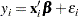where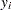is the response variable for theth observation. The quantity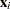is a column vector of covariates, or explanatory variables, for observationthat is known from the experimental setting and is considered to be fixed, or nonrandom. The vector of unknown coefficientsis estimated by a least squares fit to the data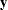. The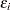are assumed to be independent, normal random variables with zero mean and constant variance. The expected value of, denoted by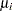, is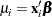While traditional linear models are used extensively in statistical data analysis, there are types of problems such as the following for which they are not appropriate.

• It might not be reasonable to assume that data are normally distributed. For example, the normal distribution (which is continuous) might not be adequate for modeling counts or measured proportions that are considered to be discrete.

• If the mean of the data is naturally restricted to a range of values, the traditional linear model might not be appropriate, since the linear predictor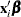can take on any value. For example, the mean of a measured proportion is between 0 and 1, but the linear predictor of the mean in a traditional linear model is not restricted to this range.

• It might not be realistic to assume that the variance of the data is constant for all observations. For example, it is not unusual to observe data where the variance increases with the mean of the data.

A generalized linear model extends the traditional linear model and is therefore applicable to a wider range of data analysis problems. A generalized linear model consists of the following components:

• The linear component is defined just as it is for traditional linear models: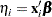• A monotonic differentiable link functiondescribes how the expected value ofis related to the linear predictor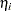: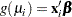• The response variablesare independent for= 1, 2,...and have a probability distribution from an exponential family. This implies that the variance of the response depends on the meanthrough a variance function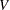: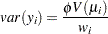where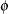is a constant and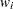is a known weight for each observation. The dispersion parameteris either known (for example, for the binomial or Poisson distribution,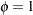) or must be estimated.

See the section Response Probability Distributions for the form of a probability distribution from the exponential family of distributions.

As in the case of traditional linear models, fitted generalized linear models can be summarized through statistics such as parameter estimates, their standard errors, and goodness-of-fit statistics. You can also make statistical inference about the parameters by using confidence intervals and hypothesis tests. However, specific inference procedures are usually based on asymptotic considerations, since exact distribution theory is not available or is not practical for all generalized linear models.